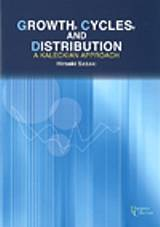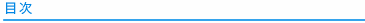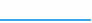ホーム ＞ 書籍詳細ページGrowth, Cycles, and Distribution

: A Kaleckian Approach

Hiroaki Sasaki

A5上製・194頁

ISBN: 9784876983940カレツキアン・モデルは、有効需要の原理に基づいて、所得分配の変化がマクロ経済変数にどのように影響するかを調査するポストケインジアン成長モデルの一種である。本書は、短期モデルだけでなく中長期のカレツキアン・モデルまで扱い、所得分配，国際競争，雇用の非正規化，金融化などが経済成長や景気循環に与える影響を理論的に分析する。Acknowledgements

Preface
1 Preliminaries
1.1 The Kaleckian model
1.1.1 Short-run model
1.1.2 Medium-run model
1.1.3 Long-run model
1.2 Short-run model
1.2.1 Adjustment of capacity utilization: Version 1
1.2.2 Determination of income distribution: Version 2

Part 1: Medium-run Kaleckian Models

2 Endogenous Technological Change, Income Distribution, and Unemployment with Inter-Class Conflict
2.1 Introduction
2.2 Basic framework of the model
2.2.1 Adjustment in the capital accumulation rate
2.2.2 Adjustment in the profit share
2.2.3 Adjustment in the growth rate of labor productivity
2.3 Existence and stability of the long-run equilibrium
2.3.1 Existence of the long-run equilibrium
2.3.2 Local stability of the long-run equilibrium
2.4 Numerical examples
2.5 Comparative statics analysis
2.6 Concluding remarks
Appendix 2.A: Determination of the equilibrium capacity utilization rate
Appendix 2.B: Determination of the equilibrium growth rate of labor productivity

3 Cyclical Growth in a Goodwin-Kalecki-Marx Model
3.1 Introduction
3.2 Basic framework of the model
3.3 Long-run equilibrium analysis
3.3.1 Existence of the long-run equilibrium
3.3.2 Local stability of the long-run equilibrium
3.3.3 Existence of closed orbits
3.3.4 Comparative statics analysis
3.4 Numerical simulations
3.4.1 Case 1 (wage-led growth): β < γ, Γ < 0
3.4.2 Case 2 (profit-led growth): β > γ, Γ < 0
3.5 Concluding remarks
Appendix 3.A: Effects of a rise in parameters on the long-run equilibrium values

Part 2: Long-run Kaleckian Models

4 Conflict, Growth, Distribution, and Employment: A Long-run Kaleckian Model
4.1 Introduction
4.2 Medium-run analysis
4.2.1 Framework of the model
4.2.2 Dynamics of the capacity utilization rate, the profit share, and the employment rate
4.2.3 Medium-run equilibrium
4.2.4 Comparative statics in the medium-run equilibrium
4.3 Long-run analysis
4.3.1 Dynamics of the normal capacity utilization rate and the expected rate of growth
4.3.2 Long-run equilibrium
4.3.3 Comparative statics in the long-run equilibrium
4.3.4 Numerical examples
4.4 Conclusion
Appendix 4.A: Endogenizing the target profit shares of workers and firms
Appendix 4.B: Derivation of the medium-run equilibrium profit share
Appendix 4.C: Local stability of the medium-run equilibrium
Appendix 4.D: Medium-run comparative statics analysis
4.4.1 A rise in A
4.4.2 A rise in s
4.4.3 A rise in n
Appendix 4.E: Derivation of equation (4.22)
Appendix 4.F: Convergence and path-dependency in the five-dimensional system with relatively small initial values (Case 1)
Appendix 4.G: Convergence and path-dependency in the five-dimensional system with relatively large initial values (Case 2)

5 Is the Long-run Equilibrium Wage-led or Profit-led? A Kaleckian Approach
5.1 Introduction
5.2 Medium-run equilibrium
5.2.1 Medium-run with Kalecki-type investment function
5.2.2 Medium-run analysis with MB-type investment function
5.2.3 Medium-run comparative statics analysis
5.3 Long-run equilibrium
5.3.1 Kalecki-type investment function
5.3.2 MB-type investment function
5.3.3 Summary
5.4 Conclusions
Appendix 5.A: Medium-run equilibrium profit share: Kalecki-type investment function
Appendix 5.B: Medium-run equilibrium profit share: MB-type investment function
Appendix 5.C: Local stability of the medium-run equilibrium: Kalecki-type investment function
Appendix 5.D: Local stability of the medium-run equilibrium: MB-type investment function
Appendix 5.E: Numerical examples in the long run: MB-type investment function

Part 3: Extended Short-run Kaleckian Models

6 The Macroeconomic Effects of the Wage Gap between Regular and Non-Regular Employment and of Minimum Wages
6.1 Introduction
6.2 The model
6.3 Characteristics of the steady state equilibrium and its stability
6.3.1 Characteristics of the steady state equilibrium
6.3.2 The stability of the steady state equilibrium
6.4 Introduction of minimum wages
6.4.1 The case of a profit-led demand regime
6.4.2 The case of a wage-led demand regime
6.5 Concluding remarks
Appendix 6.A: Introducing induced technical change
10 list of figures

7 International Competition and Distributive Class Conflict in an Open Economy Kaleckian Model
7.1 Introduction
7.2 Model
7.2.1 Dynamics of capacity utilization
7.2.2 Dynamics of the profit share
7.2.3 Dynamics of the real exchange rate
7.3 Dynamics of the model
7.4 Comparative static analysis
7.4.1 Saving rate of capitalists
7.4.2 Bargaining powers of firms and workers
7.4.3 Target value of the real exchange rate
7.4.4 Summary
7.5 Conclusions
Appendix 7.A: Stability analysis and proofs of propositions
Appendix 7.B: Numerical simulations
Appendix 7.C: Closed economy model

References
Index